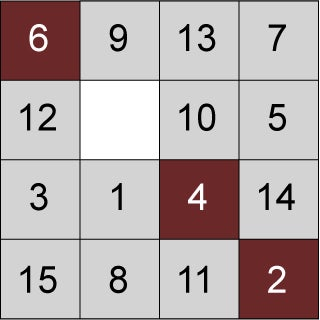6.042J | Spring 2015 | Undergraduate

# Mathematics for Computer Science

#### Course Description

This subject offers an interactive introduction to discrete mathematics oriented toward computer science and engineering. The subject coverage divides roughly into thirds:

1. Fundamental concepts of mathematics: Definitions, proofs, sets, functions, relations.
2. Discrete structures: graphs, state machines, modular …

## Course Info

##### Learning Resource Types
Online Textbook
Lecture Videos
Lecture Notes
Problem Sets
Exams6.042 serves as an introduction to discrete mathematics, probability, and mathematical thinking for computer scientists. (Image by OpenCourseWare, based on an image by Nick Matsakis.)